﻿ Analysis of Identification Accuracy of Nonlinear System Based on Volterra Model in Frequency Domain

### Analysis of Identification Accuracy of Nonlinear System Based on Volterra Model in Frequency Domain

Vitaliy Pavlenko, Viktor SperanskyyOPEN ACCESSPEER-REVIEWED

## Analysis of Identification Accuracy of Nonlinear System Based on Volterra Model in Frequency Domain

Vitaliy Pavlenko1, Viktor Speranskyy2,1ICS, Odessa National Polytechnic University, Odessa, Ukraine

2BEITI, Odessa National Polytechnic University, Odessa, Ukraine

### Abstract

The accuracy of the interpolation method for identification of nonlinear dynamical systems based on the Volterra model in the frequency domain is studied. To highlight the n–th partial component in the response of the system to the test signal the n–th partial derivative of the response using the test signal amplitude is found and its value is taken at zero. The polyharmonic signals are used as test ones. The algorithmic and software toolkit is developed for identification processes. This toolkit is used to construct the informational model of test system. The model is built as a first, second and third order amplitude–frequency characteristics and phase–frequency characteristics. The comparison of obtained characteristics with standard is given.

### At a glance: Figures

123456
Prev Next

• Pavlenko, Vitaliy, and Viktor Speranskyy. "Analysis of Identification Accuracy of Nonlinear System Based on Volterra Model in Frequency Domain." American Journal of Modeling and Optimization 1.2 (2013): 11-18.
• Pavlenko, V. , & Speranskyy, V. (2013). Analysis of Identification Accuracy of Nonlinear System Based on Volterra Model in Frequency Domain. American Journal of Modeling and Optimization, 1(2), 11-18.
• Pavlenko, Vitaliy, and Viktor Speranskyy. "Analysis of Identification Accuracy of Nonlinear System Based on Volterra Model in Frequency Domain." American Journal of Modeling and Optimization 1, no. 2 (2013): 11-18.

 Import into BibTeX Import into EndNote Import into RefMan Import into RefWorks

### 1. Introduction

It is necessary to consider technical conditions of the communication channels (CC) operation for effective data transfer. Changes in environmental conditions cause reducing the transmission data rate: in the digital CC – up to a full stop of the transmission, in analog CC – to the noise and distortion of the transmitted signals. The new methods and supporting toolkit are developing to automate the measurement of parameters and taking into account the characteristics of the CC. This toolkit allows obtaining the informational and mathematical model of such nonlinear dynamic object, as the CC , i.e. to solve the identification problem.

Modern continuous CCs are nonlinear stochastic inertial systems. The model in the form of integro–power Volterra series used to identify them [2, 3, 4, 5].

The nonlinear and dynamic properties of such system are completely characterized by a sequence of multidimensional weighting functions – Volterra kernels).

Building a model of nonlinear dynamic system in the form of a Volterra series lies in the choice of the test actions form. Also it uses the developed algorithm that allows determining the Volterra kernels and their Fourier–images for the measured responses (multidimensional amplitude–frequency characteristics (AFC) and phase–frequency characteristics (PFC)) to simulate the CC in the time or frequency domain, respectively. .

The additional research of new method of nonlinear dynamical systems identification, based on the Volterra model in the frequency domain is proposed. This method lies in n–fold differentiation of responses of the identifiable system by the amplitude of the test polyharmonic signals. The developed identification toolkit is used to build information model of the test nonlinear dynamic object in the form of the first, second and third order model. 

### 2. Volterra Models in Frequency Domain

Generally, “input–output” type ratio for nonlinear dynamical system can be presented by Volterra series [2, 3]:(1)

where x(t) and y(t) are input and output signals of system respectively;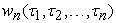-weight function or norder Volterra kernel;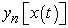-n–th partial component of object response .

In practice, Volterra series are replaced by polynomial and generally limited to several first members of the series. Identification of nonlinear dynamical system in the form of Volterra series consists of determination ofn–dimensional weighting functions wn1,…,τn) or their Fourier–images Wn(jω1,…,jωn) – n-dimensional transfer functions, accordingly to system modeling in time or frequency domain [5, 6].

Identification of nonlinear system in frequency domain consists in determination of absolute value and phase of multidimensional transfer function at given frequencies –multidimensional AFC |Wn(jω1,jω2,…,jωn)| and PFC argWn(jω1,jω2,…,jωn) which are defined by formulas:,(2)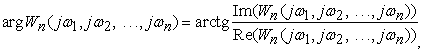where Re and Im – accordingly real and imaginary parts of a complex function of n variables.

An interpolation method of identification of the nonlinear dynamical system based on Volterra series is used [8, 9]. It is used n–fold differentiation of a target signal on parameter–amplitude a of test actions to separate the responses of the nonlinear dynamical system on partial components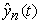.

The test signal of ax(t) type is sent to input of the system, where x(t) – any function; |a|≤1 – scale factor for n–th order partial component allocationfrom the measured response of nonlinear dynamical system. In such case it is necessary to find nth private derivative of the response on amplitude a at a=0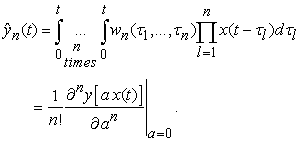(3)

Partial components of responsescan be calculated by using the test actions and procedure (3). Diagonal and subdiagonal sections of Volterra kernel are defined on basis of calculated responses.

Formulas for numerical differentiation using central differences for equidistant knots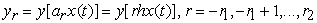with step of computational mesh on amplitude h=a  are received. Volterra kernel of the first order is defined as the first derivative at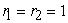or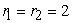accordingly(4)

Volterra kernel of the second order is defined as the second derivative ator, accordingly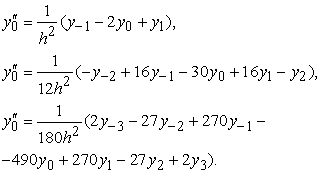(5)

Volterra kernel of the third order is defined as the third derivative ator, accordingly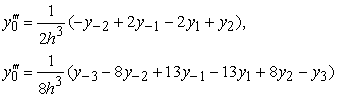(6)

where n – the order of transfer function being estimated; Al, ωl and φl – amplitude, frequency and phase of lth harmonics accordingly. It is supposed in research, that all amplitudes of Al are equal and phases φl are equal to zero.

The amplitudes of the test signals аi(k) and the corresponding coefficients ci(k) for responses are shown in Table 1, where:

k – order of the estimated Volterra kernel;

i  number of the experiment (i=1, 2, …, N);  quantity of interpolation knots, i.e. quantity of identification experiments.

Thus, the test signal can be written in complex form:(7)

Then the n–th partial component in the response of system can be represented in the following form: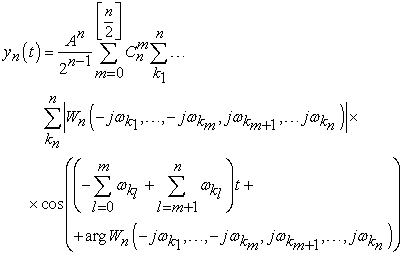(8)

heremeans function for extraction of an integer part of number.

The component with summary frequency ω1+…+ωn is selected from the response to test signal (7):(9)

In  it is defined that during determination of multidimensional transfer functions of nonlinear systems it is necessary to consider the imposed constraints on choice of the test polyharmonic signal frequencies. It provides an inequality of combination frequencies in output signal harmonics: ω1≠0, ω2≠0 and ω1≠ω2 for the second order identification procedure, and ω1≠0, ω2≠0, ω3≠0, ω1≠ω2, ω1≠ω3, ω2≠ω3, 2ω1≠ω23, 2ω2≠ω13, 2ω3≠ω12, 2ω1≠ω2–ω3, 2ω2≠ω1–ω3, 2ω3≠ω1–ω2, 2ω1≠–ω23, 2ω2≠–ω13 и 2ω3≠–ω12 for the third order identification procedure.

Given method was fully tested on a nonlinear test object (Figure 1) described by Riccati equation: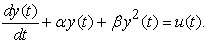(10)

Analytical expressions of AFC and PFC for the first, second and third order model were received: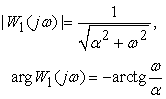(11)(12)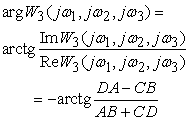(13)

where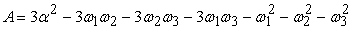;;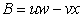;;### 3. The Techniques of Test Object Identification

The main purpose was to identify the multifrequency performances characterizing nonlinear and dynamical properties of nonlinear test object. Volterra model in the form of the second order polynomial is used. Thus, test object properties are characterized by transfer functions of W1(jω1), W2(jω1,jω2), W3(jω1,jω2,jω3) − by Fourier–images of weight functions w1(t), w2(t1, t2) and w3(t1, t2, t3).

Structure charts of identification procedure – determinations of the 1‒, 2‒ and 3–order AFC of CC are presented accordingly on Figure 2, Figure 3 and Figure 4.

Figure 2. The structure chart of identification procedure using the first order Volterra model in frequency domain, number of experiments N=4
Figure 3. The structure chart of identification procedure using the second order Volterra model in frequency domain, number of experiments N=4
Figure 4. The structure chart of identification procedure using the third order Volterra model in frequency domain, number of experiments N=4

The weighted sum is formed from received signals – responses of each group (Figure 2, Figure 3, and Figure 4). As a result the partial components of CC responses y1(t), y2(t) and y3(t) are got. For each partial component of the response the Fourier transform (the FFT is used) is calculated, and from received spectrum only an informative harmonics (which amplitudes represent values of required characteristics of the first, second and third orders AFC) are taken.

The first order AFC |W1(jω1)| and PFC argW1(jω1), where ω1 are received by extracting the harmonics with frequency f from the spectrum of the CC partial response y1(t) to the test signal x(t)=A/2(cosωt).

The second order AFC |W2(jω1,jω2)| and PFC argW2(jω1,jω2), where ω1 and ω21+Ω1, were received by extracting the harmonics with summary frequency ω12 from the spectrum of the CC partial response y2(t) to the test signal x(t)=(A/2)(cosω1t+cosω2t).

The third order AFC |W3(jω1,jω2,jω3)| and PFC argW3(jω1,jω2,jω3), where ω1=ω, ω21+Ω1, ω32+Ω2 were received by extracting the harmonics with summary frequency ω123 from the spectrum of the CC partial response y2(t) to the test signal x(t)=(A/2)(cosω1t+cosω2t+cosω3t).

The results (first, second and third order AFC and PFC) which had been received after procedure of identification are represented in Figure 5 Figure 6 and Figure 7 (number of experiments for the model N=4).

The surfaces shown on Figure 8, Figure 9, Figure 10 and 11 are built from subdiagonal cross–sections which were received separately. Ω1 was used as growing parameter of identification with different value for each cross–section in second order characteristics. Fixed value of Ω2 and growing value of Ω1 were used as parameters of identification to obtain different value for each cross–section in third order characteristics.

Figure 5. First order AFC and PFC of the test object: analytically calculated values (1), section estimation values with number of experiments for the model N=4 (2)
Figure 6. Second order AFC and PFC of the test object: analytically calculated values (1), subdiagonal cross–section values with number of experiments for the model N=4 (2), Ω1=0,01 rad/s
Figure 7. Third order AFC and PFC of the test object: analytically calculated values (1), subdiagonal cross-section values with number of experiments for the model N=6 (2), Ω1=0,01 rad/s, Ω2=0,1 rad/s

The second order surfaces for AFC and PFC which had been received after procedure of the test object identification are shown in Figure 8, and Figure 9 (number of experiments for the model N=4).

The third order surfaces for AFC and PFC which had been received after procedure of the test object identification are presented in Figure 10 and Figure 11 (number of experiments for the model N=6).

Comparison of the numerical values of identification accuracy using interpolation method and approximation one (studied earlier in ) for the test object are represented in Table 2.

Figure 8. Surface of the test object AFC built of the second order subdiagonal cross–sections received for N=4, Ω1=0,01 rad/s
Figure 9. Surface of the test object PFC built of the second order subdiagonal cross–sections received for N=4, Ω1=0,01 rad/s
Figure 10. Surface of the test object AFC built of the third order subdiagonal cross–sections received for N=6, Ω1=0,01 rad/s, Ω2=0,1 rad/s
Figure 11. Surface of the test object PFC built of the third order subdiagonal cross-sections received for N=6, Ω1=0,01 rad/s, Ω2=0,1 rad/s

### 4. Hardware-Software Tools and Technique of Radiofrequency CC Identification

Experimental research of the Ultra High Frequency range CC were done. The main purpose was the identification of multifrequency performances that characterize nonlinear and dynamical properties of the CC. Volterra model in the form of the second order polynomial is used. Thus physical CC properties are characterized by transfer functions of W1(j2πf) and W2(j2πf1,j2πf2) − by the Fourier–images of weighting functions w1 (t) and w2 (t1, t2).

Implementation of identification method on the IBM PC computer basis has been carried out using the developed software in C++ language. Such classes as CWaveRecorder, CWavePlayer, CWaveReader, CWaveWriter were used, because they allow to provide rather convenient interacting with MMAPI Windows. The software allows automating the process of the test signals forming with the given parameters (amplitudes and frequencies). Also this software allows transmitting and receiving signals through an output and input section of PC soundcard, to produce segmentation of a file with the responses to the fragments, corresponding to the CC responses being researched on test polyharmonic effects with different amplitudes.

In experimental research two identical S.P.RADIO A/S, RT2048VHF VHF–radio stations (the range of operational frequencies is 154,4−163,75MHz) and IBM PC with Creative SBLive! soundcards were used. Sequentially AFC of the first and second orders were defined. The method of identification with number of experiments N=4 was applied.

Structure charts of identification procedure – determinations of the 1‒, 2‒ and 3–order AFC of CC are presented accordingly on Figure 2, Figure 3, and Figure 4.

General scheme of a hardware–software complex of the CC identification, based on the data of input–output type experiment is presented in Figure 12.

The CC received responsesto the test signals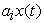, compose a group of the signals, which amount is equal to the used number of experiments N (N=4), shown in Figure 13.

In each following group the frequency of signals increases by value of chosen step. А cross–correlation was used to define the beginning of each received response. Information about the form of the test signals given in  were used.

In described experiment with use of sound card the maximum allowed amplitude was A=0,25V (defined experimentally with use of oscilloscope to take the signals without overloads). The range of frequencies was defined by the sound card pass band (20…20000Hz), and frequencies of the test signals has been chosen from this range, taking into account restrictions specified above. Here the parameters were chosen for the experiment: start frequency fs =125Hz; final frequency fe =3125Hz; a frequency change step ∆f=125Hz; to define AFC of the second order determination, an offset on frequency F1=f2f1 was increasingly growing from 201 to 3401Hz with step 100Hz.

The weighed sum is formed from received signals – responses of each group (Figure 2 and Figure 3). As a result we get partial component s of response of the CC y1(t) and y2(t). For each partial component of the response an FFT is calculated, and from received spectra only an informative harmonics (which amplitudes represents values of required characteristics of the first and second orders AFC) are taken.

The first order AFC |W1(j2πf)| is received by extracting the harmonics with frequency f from the spectrum of the partial response of the CC y1(t) to the test signal x(t)=A/2(cos2πft).

The second order AFC |W2(j2πf,j2π(f+ F1))|, where f1=f and f2=f+F1, was received by extracting the harmonics with summary frequency f1+f2 from the spectrum of the partial response of the CC y2(t) to the test signal x(t)=(A/2)(cos2πf1t+cos2πf2t).

The wavelet noise–suppression was used to smooth the output data of the experiment . The results received after digital data processing of the experimental data (wavelet “Coiflet” de–noising) for the first and second order AFC are presented in Figure 14 Figure 15, and Figure 16.

The surface shown on Figure 16 was built from subdiagonal cross–sections that has been received separately. A growing parameter of identification Δf with different value for each section was used.

Figure 15. Subdiagonal cross–sections of AFCs of the second order after wavelet “Coiflet” second level de–noising at different frequencies: 201 (1), 401 (2), 601 (3), 801 (4), 1001 (5), 1401 (6) Hz
Figure 16. Surface built of AFC crosss–sections of the second order after wavelet “Coiflet” 3rd level de–noising

### 5. Conclusions

The method based on Volterra model using polyharmonic test signals to identify nonlinear dynamical systems is analyzed. To differentiate the responses of object for partial components we use the method based on composition of linear responses combination on test signals with different amplitudes.

New values of test signals amplitudes were defined and model were validated using the test object. Excellent accuracy level for received model (with relative error no more than 10%) is achieved as in liner model so in some nonlinear ones. Given values are greatly raising the accuracy of identification in compare to amplitudes and coefficients written in . The accuracy of identification of nonlinear part of the test object growth almost 10 times and the relative error in best cases is no more than 5%. There is some problem for further researches to find the ways of identification results improvement using different number of experiments with different kernel orders.

The interpolation method of identification using the hardware methodology written in  is applied for constructing of informational Volterra model as an APC of the first and second order for UHF–band radio channel.

The received results had confirmed significant nonlinearity of the test object characteristics that leads to distortions of signals in the different type radio devices.

The received frequency characteristics of the CC in further researches will be used to decrease level of nonlinear distortions in telecommunication systems taking into account its nonlinear characteristics.

### References

  Giannakis, G.B., Serpedin, E. “A bibliography on nonlinear system identification and its applications in signal processing, communications and biomedical engineering”. Signal Processing – EURASIP, Elsevier Science B.V., 81(3), 2001, 533-580.In article  Westwick, D.T. Methods for the Identification of Multiple–Input Nonlinear Systems, Departments of Electrical Engineering and Biomedical Engineering, McGill University, Montreal, Quebec, Canada, 1995, 192-232.In article  Doyle, F.J, Pearson, R.K., Ogunnaike, B.A. Identification and Control Using Volterra Models. Published Springer Technology & Industrial Arts, 2001, 58-72.In article  Boyd, S., Jang, Y.S., Chua, L.O. “Measuring Volterra Kernels”. IEEE Trans. on Circuits and Systems, Vol. CAS-30, No.8, 1983, 571-578.In article CrossRef  Peddanarappagari, K.V., Brandt-Pearce, M. “Volterra Series Approach for Optimizing Fiber–Optic Communications System Designs”. J. Lightwave Tech., 16 (11), 1998, 2046-2055.In article CrossRef  Chang, K.F., Binh, L.N., Cambrell, G.K., Dabke, K.P. “Nonlinear frequency domain modeling of optical fibre transmission systems by Volterra series”. IOOC/OECC Conference, Sydney, July 2001, 360-362.In article PubMed  Pavlenko V.D. “Identification of Nonlinear Dynamic Systems in the Form of the Volterra Kernels on the Basis of the Data of Pulse Response Measurements”. Electronic Modeling, Vol. 32, №3, 2010, 3-18.In article  Pavlenko, V.D., Speranskyy, V.O. Identification of Nonlinear Dynamical Systems Using Volterra Model with Interpolation Method in Frequency Domain. Electrotechic and Computer Systems. № 05 (81), 2012, 229-234.In article  Schetzen, M. The Volterra and Wiener Theories of Nonlinear Systems. Wiley & Sons, New York, 1980, 321-360.In article  Pavlenko, V.D., Issa, S.I.M. “The Limitation of the choice frequencies test polyharmonic signals for the identification nonlinear system”. Proceedings of Odessa Polytechnic University, Ukraine, 1 (31), 2008, 107-113.In article  Goswami, J.G., Chan, A.K. Fundamentals of Wavelets: Theory, Algorithms, and Applications. Publishing John Wiley & Sons, Inc., 1999, 125-137.In article  Danilov, L.V., Mathanov, P.N., Philipov, E.S. The theory of nonlinear electrical circuits. Published Energoatomizdat, Leningrad, 1990, 136-148.In article  Pavlenko, V.D., Speranskyy, V.O. “Communication Channel Identification in Frequency Domain Based on the Volterra Model”. Proceedings of the International Conference on Computers, Digital Communications and Computing (ICDCC'11), Barcelona, Spain, September 15-17, 2011. Published by WSEAS Press, 2011, 218-222.In article